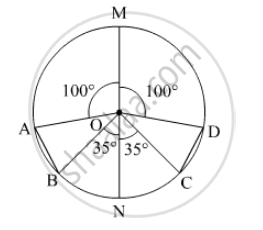SSC (English Medium) Class 8Maharashtra State Board
Share

# In the Adjoining Figure O is the Centre of the Circle Whose Diameter is Mn. Measures of Some Central Angles Are Given in the Figure. - SSC (English Medium) Class 8 - Mathematics

ConceptArcs Corresponding to the Chord of a Circle

#### Question

In the adjoining figure O is the centre of the circle whose diameter is MN. Measures of some central angles are given in the figure. hence find the following.(1) m∠ AOB and m∠ COD
(2) Show that arc AB ≌ arc CD.
(3) Show that chord AB ≌ chord CD

#### Solution

(1) MN is the diameter of the circle with centre O.

∠MOA + ∠AOB + ∠BON = 180°

⇒ 100° + ∠AOB + 35° = 180°

⇒ ∠AOB = 180° - 100° - 35° = 45°

Similarly, ∠COD = 45°

(2) Eqaual arcs subtend equal angles at the centre.

∠AOB = ∠COD = 45°

So, arc AB ≌ arc CD
(3) Corresponding chords of congruent arcs are congruent.

arc AB ≌ arc CD
So, chord AB ≌ chord CD

Is there an error in this question or solution?

#### APPEARS IN

Balbharati Solution for Balbharati Class 8 Mathematics (2019 to Current)
Chapter 17: Circle : Chord and Arc
Practice Set 17.2 | Q: 2 | Page no. 118
Solution In the Adjoining Figure O is the Centre of the Circle Whose Diameter is Mn. Measures of Some Central Angles Are Given in the Figure. Concept: Arcs Corresponding to the Chord of a Circle.
S